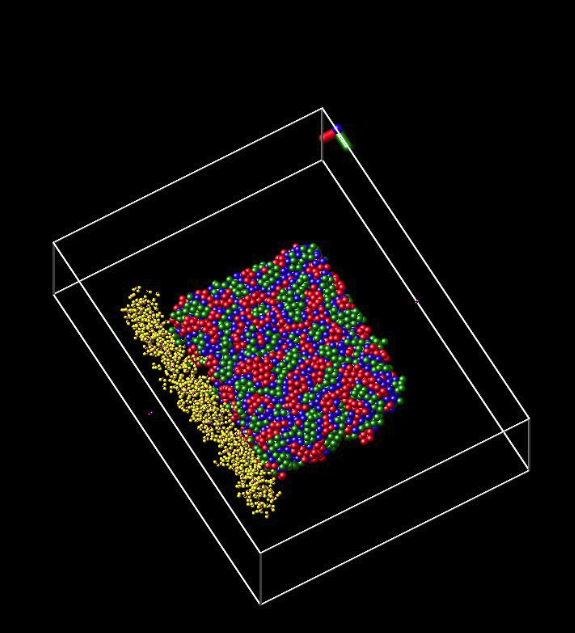# reax/c simulation stopped updation without reporting any error

Dear lammps experts,

I run a reax/c simulation between water and metal by the following 2 steps. The initial configuration may look like the attached figure.

（1）50000 timesteps NVT for the equilibration of metal only；

（2）100000 timesteps NPT reactive MD between water and metal；

However, when the NVT finished and the simulation entered NPT, it halted at 53732ed timestep without reporting any errors. It just stopped updating from the 53732ed timestep as follows:

Step = 53731 Time = 195.2 Atoms = 7534

Temp = 599.8 Press = 139 PotEng = -78.62

KinEng = 1.788 TotEng = -76.83 E_pair = -78.62

v_eb = -1.23e+06 v_ea = 3.341e+05 v_elp = -5.478e-06

v_emol = 0 v_ev = 2170 v_epen = 3.525e-12

v_ecoa = 0 v_ehb = -1712 v_et = 7.523

v_eco = 0 v_ew = 3.235e+05 v_ep = -8.017e+04

v_efi = 0 v_eqeq = 5.947e+04 c_reax = -78.62

---------------- Step 53732 ----- CPU = 1908.5160 (sec) ----------------

Step = 53732 Time = 195.5 Atoms = 7534

Temp = 600.1 Press = 139.1 PotEng = -78.62

KinEng = 1.788 TotEng = -76.83 E_pair = -78.62

v_eb = -1.23e+06 v_ea = 3.341e+05 v_elp = -5.208e-06

v_emol = 0 v_ev = 2172 v_epen = 3.63e-12

v_ecoa = 0 v_ehb = -1713 v_et = 7.582

v_eco = 0 v_ew = 3.235e+05 v_ep = -8.018e+04

v_efi = 0 v_eqeq = 5.947e+04 c_reax = -78.62

What is possible reasons for such strange phenomenon.

Any advice can be highly appreciated,